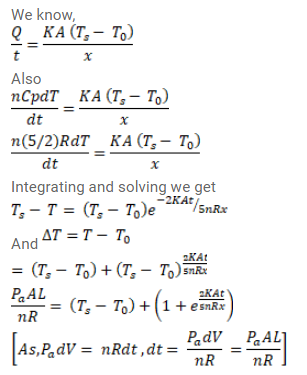Deepak Scored 45->99%ile with Bounce Back Crack Course. You can do it too!

# An amount n (in moles) of a monatomic gas at an initial temperature

Question:

An amount $n$ (in moles) of a monatomic gas at an initial temperature $T_{0}$ is enclosed in a cylindrical vessel fitted with a light piston. The surrounding air has a temperature $T_{s}\left(>T_{0}\right)$ and the atmospheric pressure is $p_{a}$. Heat may be conducted between the surrounding and the gas through the bottom of the cylinder. The bottom has a surface area $A$, thickness $x$ and thermal conductivity K. Assuming all changes to be slow, find the distance moved by the piston in time $t$.

Solution:$L=\frac{n R}{P_{a} A}\left(T_{s}-T_{0}\right)+\left(1-e^{\frac{-2 K A t}{5 n R x}}\right)$